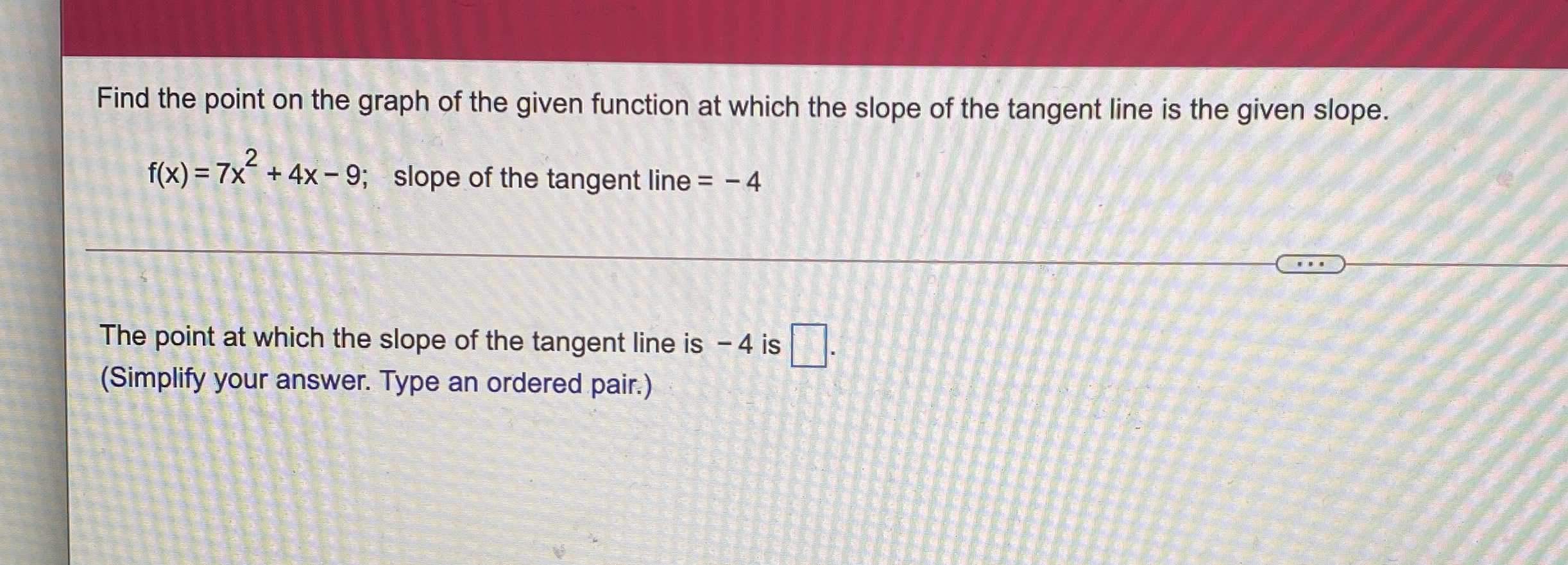### Still have math questions?

Algebra
QuestionFind the point on the graph of the given function at which the slope of the tangent line is the given slope.

$$f ( x ) = 7 x ^ { 2 } + 4 x - 9 ;$$ slope of the tangent line $$= - 4$$

The point at which the slope of the tangent line is $$- 4$$ is (    ).

$$(- \frac{4}{7},- 9)$$